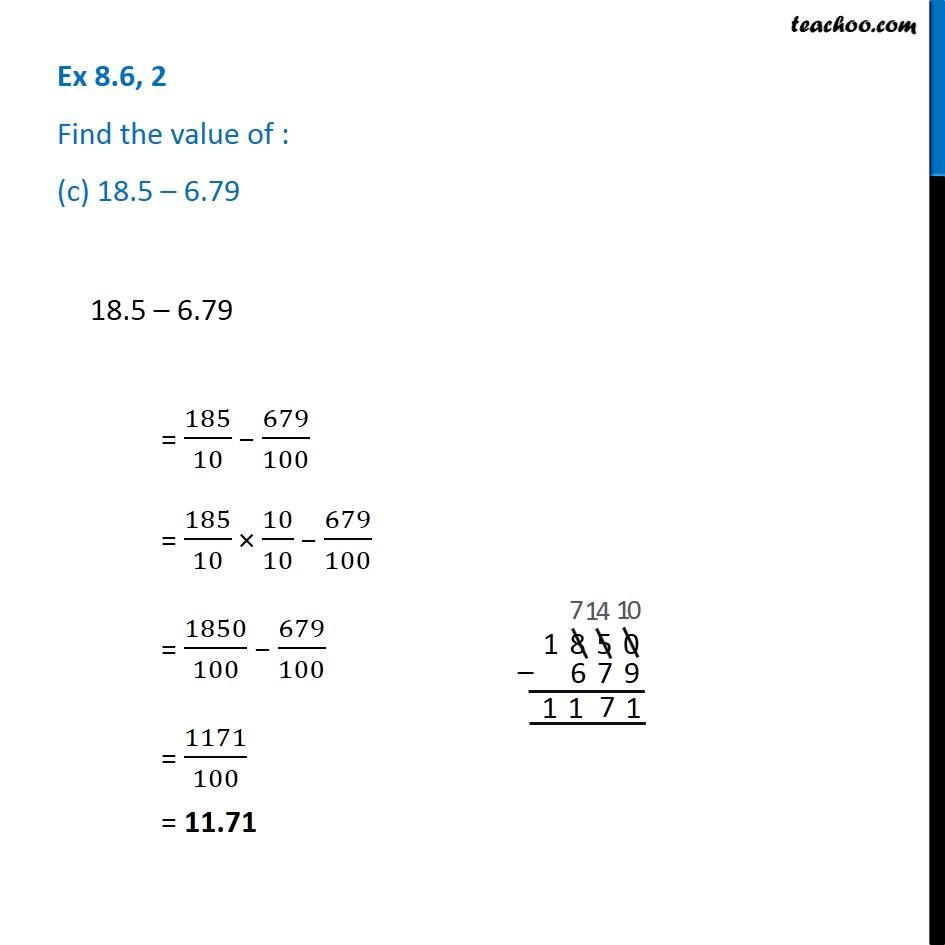1. Chapter 8 Class 6 Decimals
2. Serial order wise
3. Ex 8.6

Transcript

Ex 8.6, 2 Find the value of : (c) 18.5 – 6.7918.5 – 6.79 = 185/10 − 679/100 = 185/10 × 10/10 − 679/100 = 1850/100 − 679/100 = 1171/100 = 11.71